Notes for Level I CFA® Program

R57 Basics of Derivative Pricing and Valuation

4.2. Binomial Valuation of OptionsWatch Video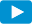This section deals with the payoff of an option. The payoff of an option depends on the value of the underlying at expiration, which cannot be known with certainty ahead of time. What matters from an option’s perspective is whether it expires in- or out-of-the money, i.e. whether the stock price was above or below the strike price at expiration.

The binomial model is a simple model for valuing options based on only two possible outcomes for a stock’s movement: going up and going down.
Π and 1- π are called the synthetic probabilities; they represent the weighted average of producing the next to possible call values, a type of expected future value. By discounting the future expected call values at the risk-free rate, we can get the current call value.

Risk-neutral probability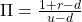where:
r = risk-free rate
u = up factor; d = down factor

Let us do a numerical example to calculate the call price using a 1 – period binomial model now. Data already given to us is in normal font, while the calculated ones are in bold.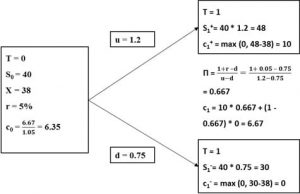The value of the call option at time 0 using the 1-period binomial model is 6.35.

Call price using the binomial model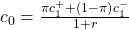where:

π = risk-neutral probability

r = risk-free rate

c0 = price of call option at time 0

Some important points about the binomial pricing model:

1. Volatility of the underlying: The volatility of the underlying is important in determining the value of the option. It is shown in the difference between S1+ and S1. The higher the volatility, the greater the difference between the stock prices S1+ and S1,and c1+ and c1.
2. The probabilities of the up and down moves do not appear in the formula.
3. Instead, what is used is the synthetic or pseudo probability, π, to determine the expected future value.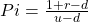4. The formula for calculating c0is the expected future value discounted at the risk-free rate.

Binomial Value of Put Options

What is the value of the put option using the same principle discussed in the previous section?

Assume the following data is given:

S0 = 40; u = 1.2; d= 0.75; X = 38; r = 5%; p0 =?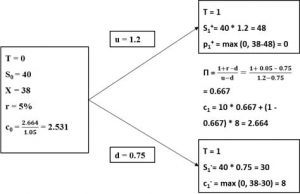The value of the put option at time 0 using the 1-period binomial model is 2.531.